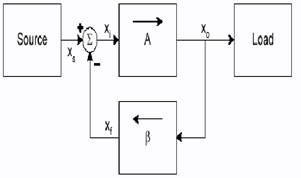EXPT NO:- 4

Title:- Current-Series feedback amplifier.

Aim:- Configure the circuit build in experiment no. 3 for Current series feedback topology and

compare practical results with theoretical analysis.

Pre-requisites:- Concept of Negative feedback

Generalizes feedback system

Equipments:- DC Power supply, Function generator, CRO, Resistors, Transistors, Capacitors,

connecting wires etc.

Theory:-

Negative Feedback -

Negative feedback is produced by feeding a portion of an amplifier output back to the input and applying the feedback quantity in opposition to the input. The result is stabilized gain, extended bandwidth, reduced noise and distortion and modified input and output impedances.

A basic configuration of feedback system is as shown in fig.Fig. a) Basic configuration of feedback system

This is a signal-flow diagram, and the quantities X represent either voltage or current signals.

The open-loop amplifier has a gain A; thus its output Xo is related to the input Xi by

Xo = A Xi (1)

The output Xo is fed to the load as well as to a feedback network, which produces a sample of the output. This sample Xf is related to Xo by the feedback factor Î²

Xf = Î² Xo (2)

The feedback signal Xf is subtracted from the source signal Xs to produce the signal Xi ,

Xi = Xs â€“ Xf (3)

So the negative feedback reduces the signal that appears at the input of the basic amplifier.

The gain of the feedback amplifier can be obtained by from Eqs. 1-3 :

Af =(Xo/Xs) = (AXi)/(Xi+Xf) = (AXi)/(Xi+ Î² AXi)

Af=A/(1+A Î²)

Where , Af =loop gain.

1 + A Î² =amount of feedback.

In many circuits, the loop gain AB is large, A Î² >> 1, it follows Af= 1/ Î²

The gain of the feedback amplifier is almost entirely determined by the feedback network.

Basic feedback topologies -

Based on the quantity to be amplified (voltage or current) and on the desired form of output (voltage or current), amplifiers can be classified into four categories.

a) voltage-series topology

b) current-shunt topology

c) current-series topology

d) voltage-shunt topology.

Current-Series feedback amplifier :

The configuration of an ideal current series feedback amplifier is as shown in fig.b)Fig. b) Current-series feedback amplifier

The circuit consists of a basic transconductance amplifier with open loop transconductance gain Gm. The feedback network samples the output current (Io) and produces a feedback voltage( Vf), which is in series with the input signal voltage (Vs).

The input impedance and output impedance of current series feedback amplifier increases as compared to the basic tranconductance amplifier. Current series feedback stabilizes transconductance gain(Gm) of amplifier.

Procedure:-

a) Connect the circuit as shown in fig.c)

b) Apply signal source Vs =20mVp-p, 1KHz and measure output voltage Vo.

c) Connect 1K resistor in series with input signal Vs and measure voltage Vb across 1k resistor.

Calculate Ii=(Vs-Vb)/1K

d) Calculate Transconductance gain (Gm) = Vo/Ii

e) Measure output Voâ€™ with 1k resistor in series with Vs and calculate

Ri=Voâ€™/(Vo-Voâ€™) X 1k.

f) Disconnect 1K and Connect 10k resistor across the output and measure output Voâ€™â€™. Calculate

Ro=[(Vo-Voâ€™â€™)/Voâ€™â€™] X 10k.

g) Calculate Vo/âˆš2 . Measure lower cut of frequency(FL)and higher cut off frequency(FH) at which

output become Vo/âˆš2. Calculate bandwith = FH -FL

h) Compare the practical results with theoretical results.

Observation Table:

 Sr. No. Quntity Measured values 1 Vo â€¦â€¦ V 2 Vb â€¦â€¦. V 2 Voâ€™ â€¦â€¦ V 3 Voâ€™â€™ â€¦â€¦.mV 4 FL â€¦ Hz 5 FH â€¦â€¦..Hz

Calculations :

I] Practical Calculations â€“

II] Theorotical calculations -

Result:-

Current-series Feedback :

 Sr. No. Parameter Without Feedback With Feedback Practical Theorotical 1 Transconductance Gain (Gm) 2 Input Impedance (Ri) 3 Output impedance (Ro) 4 Bandwidth